Next: Heun Predictor - Corrector Up: How To Implement a Previous: Results

# Volume Calculation (Gauss Theorem)

A mathematical expression which describe Gauss theorem can be written in following form: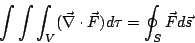(7)

Where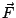is a vector field,is whole body volume and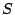is body surface. Let us rewrite it in a case of two dimensional object: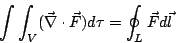(8)

Whereis body field,is any vector field andis body edge. If we assume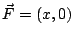then because of: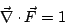(9)

and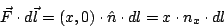(10)

we will get a simple expression which describe body field value (our "volume" calculated in the code):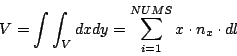(11)Next: Heun Predictor - Corrector Up: How To Implement a Previous: Results
Maciej Matyka 2004-03-30